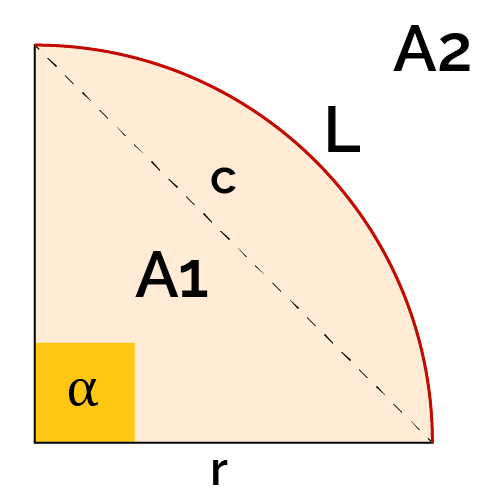# Quarter Circle Area Calculator

Created by Łucja Zaborowska, MD, PhD candidate
Reviewed by Gabriela Diaz
Last updated: Sep 23, 2022

You've finally reached the quarter circle area calculator; this tool offers everything you need to know about the area of a quarter circle and its formula. Keep on reading to find out more! ⤵️

We'll teach you:

• How to find the area of a quarter circle;
• How to use the 1/4 circle formula and the quarter circle perimeter equation;
• What's the chord of the 4th part of a circle; and
• How to calculate the quarter arc.

💡 Looking for different types of circles? Try other tools from the sector area calculator series:

## How to calculate the arc of a quarter circle?

The arc of a quarter circle equals 1/4 of a perimeter of the entire circle. If we want to calculate it, we need to divide the mentioned parameter by 4.

The entire circle perimeter = 2 × π × r²

The arc of a quarter circle = (π × r²)/ 2

It's that simple, isn't it?

## How to calculate the chord of a quarter circle?

Calculating the chord of a quarter circle is a little bit difficult since it encompasses a conversion of degrees to radians. Let's begin with the equation itself:

Chord = 2 × r × sin(1.57/ 2)
Chord = 2 × r × sin(0.79)

Where r stands for radius of the circle, and sine is given in radians.

How do we know it? The quarter circle contains a 90° angle equal to 1.57 radians.

## How to calculate the quarter circle perimeter?

1. The quarter circle perimeter consists of two components:

2 × r

• Arc of the quarter circle.

(π × r²)/ 2

1. Let's combine them together:

The quarter circle perimeter = (2 × r) + (π × r²/ 2)

## How to calculate the area of a quarter circle?

Let's make it nice and simple!

The quarter circle equals 1/4 of the entire circle; in order to calculate it, we need to divide the are of the whole circle by 4:

Whole circle area = π × r²

Quarter circle area = (π × r²)/ 4

r - the radius of the circle, equal to half of the diameter.

## How to use the quarter circle area calculator?

It just couldn't be easier - and we sure mean it!

And well.. that's it. Enjoy your results. 🎉

## FAQ

### How many quarter circles equal a whole circle?

4 quarter circles equal a whole circle.

That's why, if you want to compute the area of a quarter circle, you need to divide the area of a whole circle by 4.

Quarter circle area = Full circle area/ 4

### How to calculate the area of a quarter circle with radius = 2?

1. It's pretty effortless - if we know that the radius = 2, and π= 3.14, we just need to follow the equation below:

Quarter circle area = (π × r²)/ 4

Quarter circle area = (π × 2²)/ 4

Quarter circle area = (π × 4)/ 4

Quarter circle area = π

1. We already know the answer: the quarter circle area with the radius of 2 equals π!
Łucja Zaborowska, MD, PhD candidatein
Quarter area (A1)
in²
Spare area (A2)
in²
Quarter arc (L)
in
Perimeter (p)
in
Chord (c)
in
People also viewed…

### Condition number

The condition number calculator finds the condition number of a matrix. It supports the use of popular matrix norms and can find a 2×2 or 3×3 matrix's condition number.

### Grams to cups

The grams to cups calculator converts between cups and grams. You can choose between 20 different popular kitchen ingredients or directly type in the product density.